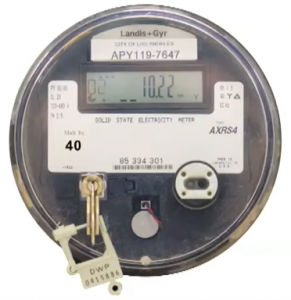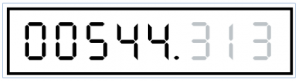## How to read an energy meter?

Energy meters calculate the amount of energy used in units called kilowatt-hour (kWh). Traditional energy meter devices use small dials. These dials show a digit and that digit represents a number of the total amount of energy consumed. To properly read the value, it is useful to write down the digits just passed by the pointer of each dial from right to left. Starting from the right then, the first dial is the unity digit. The one to the left of it is the tens digit. Then, the next dials to the left are respectively the ones representing the hundreds and the thousands of the number being the current meter’s reading. In the example below, the reading of the energy meter is 7830 kWh.It is important to start the reading from the right side to not miss out on any significant digits at the end of the total number value. Also, the dial which is the furthest one to the right is the one that causes the movement of all the other dials. When it makes a full rotation, then the one to the left of it moves from 0 to 1. When that one makes a full revolution as the effect of the furthest one to the right moving, it effectively makes the third one from the right to move as well. This process continues all the way to the dial on the left, being the last significant digit of the total energy meter’s reading.

### Modern Devices

More modern energy meters with the digital display may require the value shown on it to be multiplied by a constant. In such a case, the value of the constant is shown on the device’s enclosure. The consumed energy amount is then the value on the energy meter’s screen (10.22) times the constant (40) which results in 408.8-kilowatt-hours.Digital energy meters with just the display and without any additional constants or any complexity in their value reading are becoming more common. In the case of these meters, a customer is required only to read out the value shown on the screen from left to right ignoring the digits shown after the decimal point (usually indicated in red). An example of such a display is presented below. Its proper reading is 544-kilowatt hours.FIND OUT WHAT SMART APPLIANCES CAN SAVE YOU THE MOST MONEY